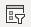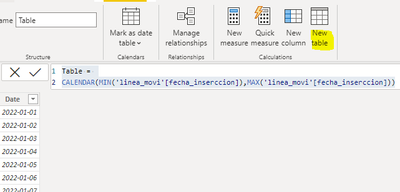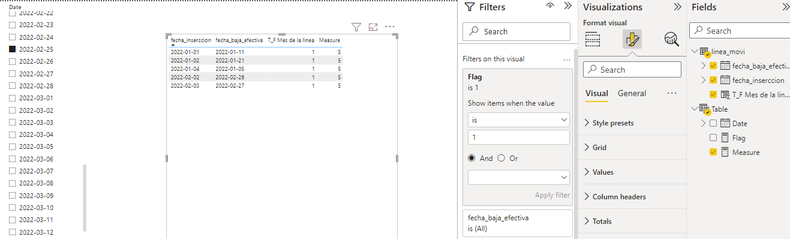cancel
Showing results for
Did you mean:Frequent Visitor

## Total by counting all records from a column if the cell contains 1

Hello,

I have created a column in a table writing 1 or 0 depending on the month and the year when the line was created and deleted.

If the month and year when the line was created is the same than when the line wsa deleted, I will write "1". Otherwise, I´ll write "0'

``````T_F Mes de la linea =
if(
MONTH(linea_movil[fecha_inserccion]) = MONTH(linea_movil[fecha_baja_efectiva])&&
YEAR(linea_movil[fecha_inserccion]) = YEAR(linea_movil[fecha_baja_efectiva]),
1,0)``````

I am trying to count from the column True_False I´ve created before all lines with value=1:

``````Total cells true =
CALCULATE (
COUNTROWS (lines) ,
lines[True_False] IN {1})``````

However, I want to count only records with lines[true_false]=1 AND by filtering with the date column (dateCreated).

If I create a date filter on the visualization withand I chose a month by clicking the filter menu, the measure I´ve created is not filtering me by date.

It shows me the total of records=1 instead of filter all records=1 by counting only the month that I´ve choose in the filter.

How can I manage it?

1 ACCEPTED SOLUTIONCommunity Support

Hi  @fguijarrob ,

I created some data:Here are the steps you can follow：

1. Create calculated table.

``````Table =
CALENDAR(MIN('linea_movi'[fecha_inserccion]),MAX('linea_movi'[fecha_inserccion]))``````2. Create measure.

``````Flag =
var _min=MINX(ALLSELECTED('Table'),[Date])
var _max=MAXX(ALLSELECTED('Table'),[Date])
RETURN
IF(
MAX('linea_movi'[fecha_inserccion])>=_min&&MAX('linea_movi'[fecha_inserccion])<=_max&&MAX('linea_movi'[T_F Mes de la linea])=1
,1,0)``````
``````Measure =
COUNTX(ALLSELECTED('linea_movi'),[T_F Mes de la linea])``````

3. Place [Flag]in Filters, set is=1, apply filter.4. Result:Total by counting all records from a column if the cell contains 1.pbix

Best Regards,

Liu Yang

If this post helps, then please consider Accept it as the solution to help the other members find it more quicklyCommunity Support

Hi  @fguijarrob ,

I created some data:Here are the steps you can follow：

1. Create calculated table.

``````Table =
CALENDAR(MIN('linea_movi'[fecha_inserccion]),MAX('linea_movi'[fecha_inserccion]))``````2. Create measure.

``````Flag =
var _min=MINX(ALLSELECTED('Table'),[Date])
var _max=MAXX(ALLSELECTED('Table'),[Date])
RETURN
IF(
MAX('linea_movi'[fecha_inserccion])>=_min&&MAX('linea_movi'[fecha_inserccion])<=_max&&MAX('linea_movi'[T_F Mes de la linea])=1
,1,0)``````
``````Measure =
COUNTX(ALLSELECTED('linea_movi'),[T_F Mes de la linea])``````

3. Place [Flag]in Filters, set is=1, apply filter.4. Result:Total by counting all records from a column if the cell contains 1.pbix

Best Regards,

Liu Yang

If this post helps, then please consider Accept it as the solution to help the other members find it more quickly